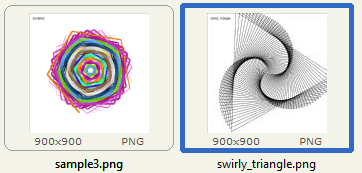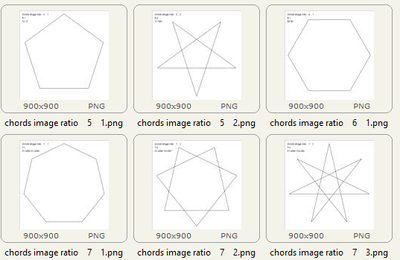## Fun with SG turtles in DS2.

Find attached a Proc DS2 package and sample usage code for simple turtle graphics.  The package takes turtle instructions issued through DS2 package instances and translates them to source code invocations of macros in SG Annotation Macro Dictionary which are stored in a data set.  The example code draws some images, and writes the generated source code to a file that is included back.Demo code is in the Spoiler because the message board would not accept it as an attachment.

Spoiler
```%* determine folder that program is in;

%let program_path = %sysfunc(transtrn(%sysget(sas_execfilepath),%sysget(sas_execfilename),));

%include "&program_path.DS2SGTI - package source.sas";

%let image_viewer = C:\Users\Richard\FSViewer74\FSViewer.exe;
%let image_folder = D:\TurtleOutput;

options source;

%if not %sysfunc(cexist(work.sasmacr.sganno.macro)) %then %do;
%sganno

%* create template for playing the annotations;
ods path(prepend) work.templat(update);
proc template;
define statgraph DS2GTI;
begingraph;
layout overlay;
annotate;            %* specify the ANNOTATE GTL statement ;
endlayout;
endgraph;
end;
run;
%end;

%macro break;
%abort cancel;
%mend;

%macro fdelete (filename);
filename _file "&filename";
%let rc = %sysfunc(fdelete(_file));
%put NOTE: %sysfunc(sysmsg());
filename _file;
%mend;

options ls=250;

ods html close;
ods listing;

proc ds2;
* A data program;
data _null_;
* global variables;

declare char(41) statements_table;       /* table that will collect the generated statements */
declare varchar(400) write_options;      /* options for %sgtext statements that will be generated */
declare varchar(300) path;               /* path where image files will be written */

declare package math math();

method init();
* global assignments for use in other methods;
statements_table = 'work.turtle_statements';
write_options = 'anchor="left", textsize=14, textfont="Albany AMT"';

/*
* NOTE:
*   DS2 has no methods for interacting with the macro environment at run-time.
*   The closest one can get is to resolve a macro symbol at source code submission time
*/
path = %str(%'&image_folder%');
end;

method sample1();
declare package canvas c;
declare package turtle t;

c = _new_ canvas(900,900, statements_table, path, 'sample1');
t = _new_ turtle(c);

put statements_table=;

t.down();

t.up();
t.setxy (-425,410);
t.down();
t.write(c.filename(), write_options);

c.close();
c.delete();
end;

method sample2();
declare package canvas c;
declare package turtle t;

declare int index;

c = _new_ canvas(900,900, statements_table, path, 'sample2');
t = _new_ turtle(c);

t.down();
do index = 1 to 96;
t.left(90);
end;

t.up();
t.setxy (-425,410);
t.down();
t.write(c.filename(), write_options);

t.delete();
c.close();
c.delete();
end;

method sample3();
declare package canvas c;
declare package turtle t;

declare int index r g b;
declare double theta;

c = _new_ canvas(900,900, statements_table, path, 'sample3');
t = _new_ turtle(c);
t.down();

r = 50;
g = 100;
b = 150;

do index = 1 to 350;
r = mod(r+2,256);
g = mod(g+3,256);
b = mod(b+4,256);

t.color(c.rgb_to_color(r,g,b));

t.left(70);

theta = constant('pi') + 8 * constant('pi') * index / 150;

t.size(8 + 7 * cos(theta));
end;

t.up();
t.setxy (-425,410);
t.down();
t.write(c.filename(), write_options);

t.delete();
c.close();
c.delete();
end;

method chord_image_for_ratio(double radius, double p, double q);
declare double angle chord_angle chord_length outside dstep;

declare package canvas c;
declare package turtle t;

c = _new_ canvas(900,900, statements_table, 'chords_image_for_ratio_anno', path, 'chords image ratio '||put(p,4.)||' '||put(q,4.));
t = _new_ turtle(c);

angle = 360. / p;
chord_angle = q * angle;

outside = 360. * q / p;

t.up();
t.setxy(
);
t.down();

do dstep = 1 to p;
t.left(outside);
end;

t.up(); t.setxy (-425,410); t.down(); t.write(c.filename(), write_options);
t.up(); t.setxy (-425,375); t.down(); t.write(p||':'||q, write_options);
t.up(); t.setxy (-425,340); t.down(); t.write(strip(put(angle,best7.)) || ' ' || strip(put(outside,best7.)), write_options);

t.delete();
c.close();
c.delete();
end;

method chord_images_for_ratios(int minp, int maxp);
declare int p1 q1 p q;
declare char(1) flag1 flag2;

do p1 = minp to maxp;
do q1 = 1 to maxp/2;
math.rational ( 1.* p1 / q1, 1000, p, q );

flag1 = ifc (p < p1, '*', ' ');
flag2 = ifc (p < 2*q,'#', ' ');

* put p1=3. q1=3. p=3. q=3. ' ' flag1 ' ' flag2;

if p < p1 then continue;
if p < 2*q then continue;

end;
end;
end;

declare double outside nangles angle chord_angle chord_length dstep;
declare int p q steps;

declare package canvas c;
declare package turtle t;

outside = 180. - theta;
nangles = 360. / outside;

math.rational ( nangles, 1000, p, q );

steps = p;

angle = 360. / p;

c = _new_ canvas(900,900, statements_table, 'chords_image_for_angle_anno', path, 'chords image angle '||put(theta,6.2));
t = _new_ turtle(c);

/*    * draw outline;*/

chord_angle = q * angle;

t.up();
t.setxy(
);
t.down();

do dstep = 1 to steps;
t.left(outside);
end;

t.up(); t.setxy (-425,410); t.down(); t.write(c.filename(), write_options);
t.up(); t.setxy (-425,375); t.down(); t.write(p||' '||q, write_options);
t.up(); t.setxy (-425,340); t.down(); t.write(angle, write_options);
t.up(); t.setxy (-425,305); t.down(); t.write(theta, write_options);

c.close();
c.delete();
end;

method chord_images_for_angles(double mintheta, double maxtheta, double delta);

do theta = mintheta to maxtheta by delta;
end;
end;

method swirly_triangle();
declare package canvas c;
declare package turtle t;

declare int index;

c = _new_ canvas(900,900, statements_table, path, 'swirly_triangle');
t = _new_ turtle(c);

t.down();
do index = 1 to 150;
t.left(121);
end;

t.up();
t.setxy (-425,410);
t.down();
t.write(c.filename(), write_options);

t.delete();
c.close();
c.delete();
end;

method run();
* rebuild statements table;
sqlexec ('drop   table ' || statements_table || ' force');
sqlexec ('create table ' || statements_table || ' (statement char(400))');

/*      sample1();*/
/*      sample2();*/
sample3();

/*      chord_images_for_angles(7, 10, 1);*/
/*      chord_images_for_ratios(5, 23);*/

swirly_triangle();
end;

enddata;
run;
quit;

%let syslast = work.turtle_statements;

options source xmin noxwait noxsync;
options nosource2;

filename stmt_pgm temp;
data _null_;
set &syslast end=last;
file stmt_pgm;
put statement;
if last then call execute ('%nrstr(%include stmt_pgm;)');
run;

filename stmt_pgm;

options noxwait noxmin noxsync;

/* %sysexec "&image_viewer" "&image_folder.\swirly_triangle.png"; */
```

Packages and methods:

• Math
• rational()
• Pen
• up()
• down()
• isDown()
• isUp()
• color()
• size()
• Canvas
• translate()
• rgb_to_color()
• setpen()
• line()
• arc()
• filename()
• write()  /* text */
• close()
• Turtle
• log()
• retreat()
• right()
• left()
• setxy()
• setx()
• sety()
• getx()
• gety()
• home()
• arc()
• dot()
• down()
• up()
• color()
• size()
• write()

The sources are named DS2SGTI for "DS2 Statistical Graphics Turtle Interface".

Back story:

The olden days of SAS/GRAPH provided the DATA Step Graphics Interface (DSGI),  a variety of functions that mimicked an implementation of the ISO Graphic Kernel Standard (GKS).   DSGI documentation went dark in SAS 9.4, and DSGI I presume is in a deprecated state.

At one point I had written Proc FCMP function adapters to the DSGI functions, in the hopes they could be called from Proc DS2.  Turns out the DSGI .dll that holds all those functions is not accessible to DS2 code, even when called through a FCMP function adapter.  I presume this is related to how DS2 programs can run in CAS which wouldn't have the DSGI functions available.

SAS/GRAPH Graph Template Language (GTL) is the new game in town, but there is no equivalent functional interface like DSGI was.  I suppose if there were one it would be called SGDSGI or DSGTLI.

Discussion stats
• 0 replies
• 371 views
• 2 likes
• 1 in conversation